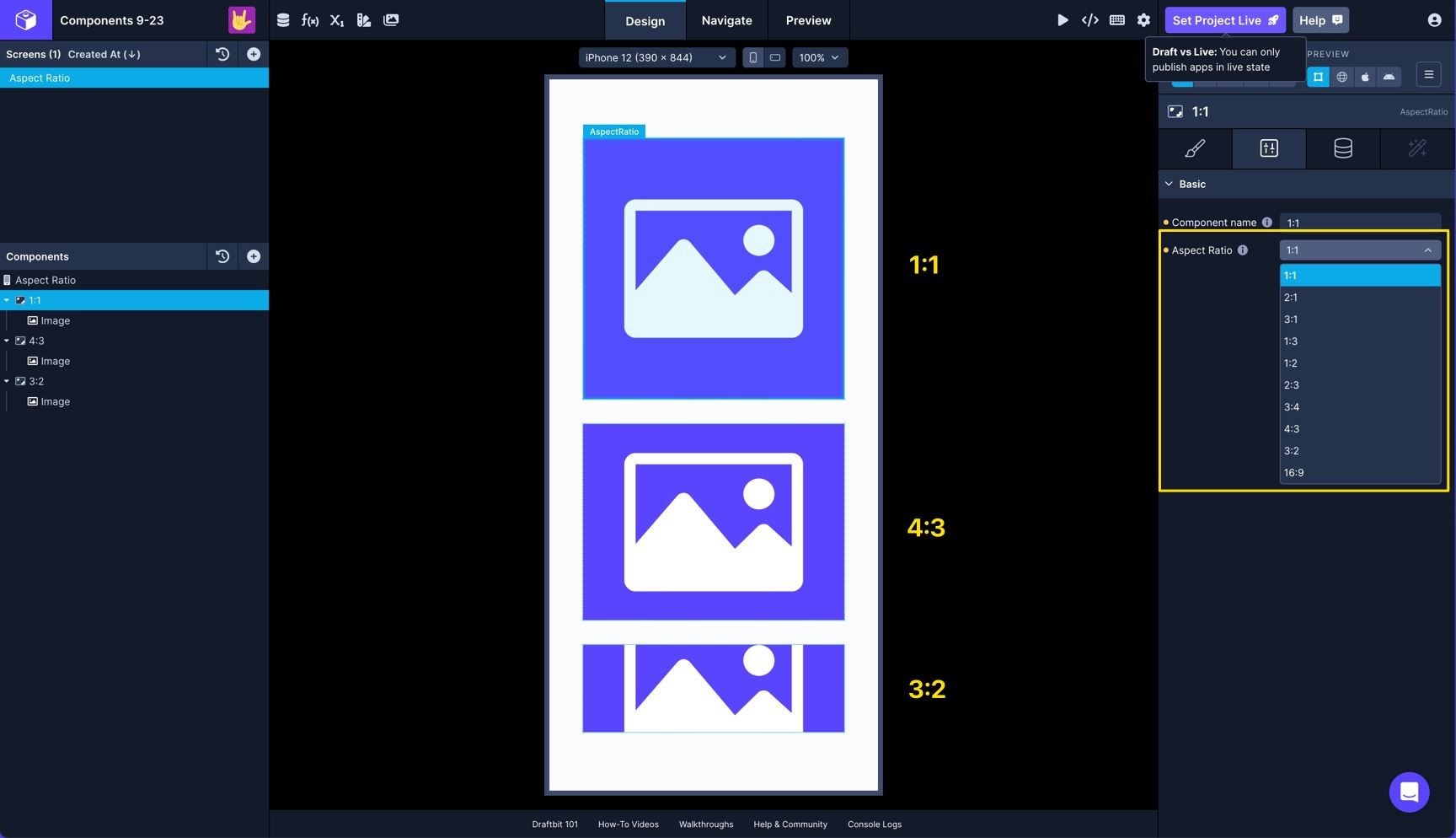# Aspect Ratio

The Aspect Ratio component constrains the dimensions of it's child(ren) to the selected aspect ratio## How to use

The Aspect Ratio components is similar to a standard View. You can nest a component, such as an Image, inside Aspect Ratio and have the image constrained by the ratio that you select.

For example, selecting a ratio of `1:1` would create a square where the length and height are proportionally equal. A ratio of `3:1` means the length is 3 times the height. A ratio of `4:3` would mean the height is 3/4 of the width.

## Configuration Properties

Component nameThe name of the component as it appears in the Screen's component tree
Aspect RatioAspect ratio of the view